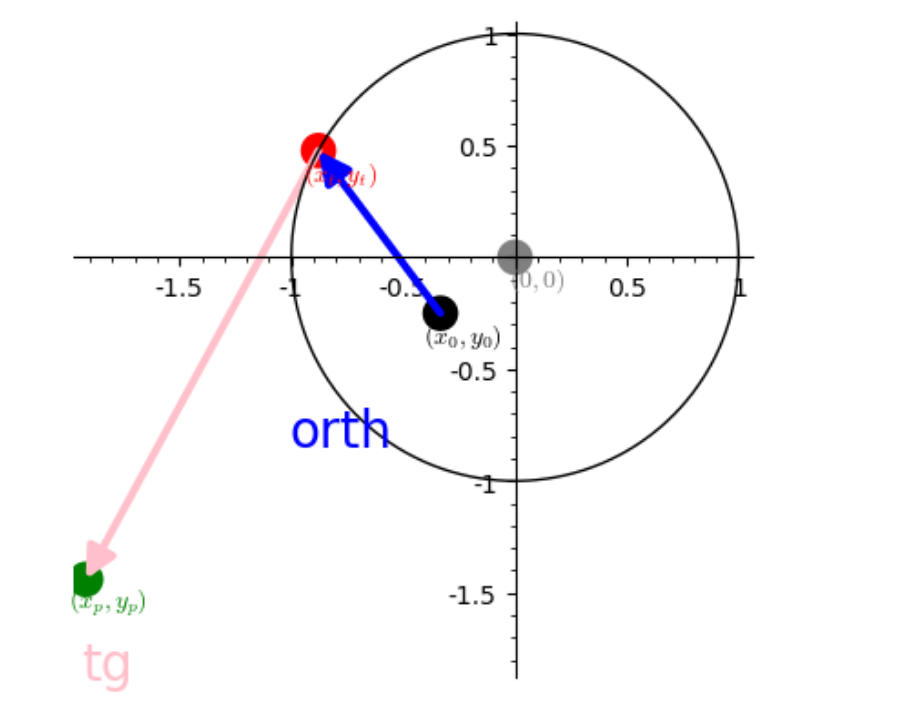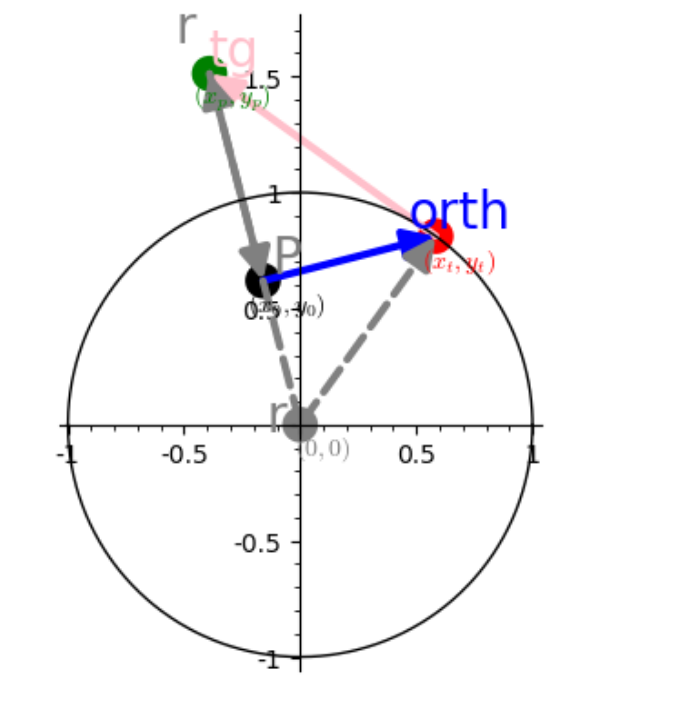# solve() need solution pre-chewed

HI

why do i have to square the two terms of the equation so that solve gives me the solution?

why it does not alow him to do square both sides ?

vL=['x','y','r','x_0','y_0','x_p','y_p','x_t','y_t','atg','btg','alpha']
varL=var(vL)
x_0Num=-1/3 ;y_0Num=-1/4 ;rNum=1

numL=[x_0==x_0Num ,y_0==y_0Num ,r==rNum]

assume(x,'real')
assume(abs(x_0)<1)
assume(abs(y_0)<1)
assume(r>=1)

C_u(x)= sqrt(r^2-x^2)
C_d(x)= -sqrt(r^2-x^2)

delta_p(x)=-x_0/y_0*x + y_0+(x_0^2)/y_0 #perpendicular to circle radial delta_0
assume(delta_p>0)
# numerical solution
SupNum=solve((C_u(x)==delta_p(x)).substitute(numL), x, to_poly_solve=True)

# literal solutions which do not work
SupLit1=solve(C_u(x)== delta_p(x), x, to_poly_solve=True)
SupLit2=solve(C_u(x)== delta_p(x), x,  algorithm='sympy')

# literal solutions ok
SupLit3=solve(C_u(x)^2== (delta_p(x))^2, x,  algorithm='sympy')
SupLit4=solve(C_u(x)^2== (delta_p(x))^2, x, to_poly_solve=True)

show("Sup num : ",SupNum)
show("Sup lit 1 : ", LatexExpr(r" \huge \color{red}  {?? }"),SupLit1)
show("Sup lit 2 : ", LatexExpr(r" \huge \color{red}  {?? }"),SupLit2)
show("Sup lit 3 : ",SupLit3, LatexExpr(r" \huge \color{green}  {OK }"))
show("Sup lit 4 : ",SupLit4, LatexExpr(r" \huge \color{green}  {OK }"))projection in the other side is easy, but unfortunately the projection matrix is not invertible.

pointInsideCircle=False # projection from outside circle to intside

# alpha is angle between radial vector([(x_0,y_0),(0,0) ]) and tangente line from x_0,y_0 to circle
# (the sides  V_R,V_tg,r form a rectangle triangle)
sin(alpha)=r/sqrt(x_0^2+y_0^2)
cos(alpha)=sqrt(1-sin(alpha)^2)
# we want it directed to the center of the circle so it is multiplyed by -1
V_R0=matrix(SR,[x_0,y_0]).transpose()*-1
#reduction factor because (||V_R||*cos(alpha)=||V_tg||)
reductFactor=cos(alpha)
# rotation matrix and shrink to get tangent vector V_tg
rotM=matrix(SR,[[cos(alpha),-sin(alpha)],[sin(alpha),cos(alpha)]])*reductFactor
# projection Matrix on radial Vector
projectOnRadialVectorM=matrix(SR,[(x_0^2/(x_0^2 + y_0^2), x_0*y_0/(x_0^2 + y_0^2)),
(x_0*y_0/(x_0^2 + y_0^2), y_0^2/(x_0^2 + y_0^2))])

# V_tg is the tangent vector
V_tg=rotM*V_R0

# vector ([[0,0],[x_t,y_t]) from (0,0) to tangent point
V_r=-v_R0+V_tg

# now project the tangent vector on radial Vector

# projected point ProjectPt of [x_0,y_0]= projected vector - radial vector [[x_0,y_0][0,0]
ProjectPt=V_p-V_R

edit retag close merge delete